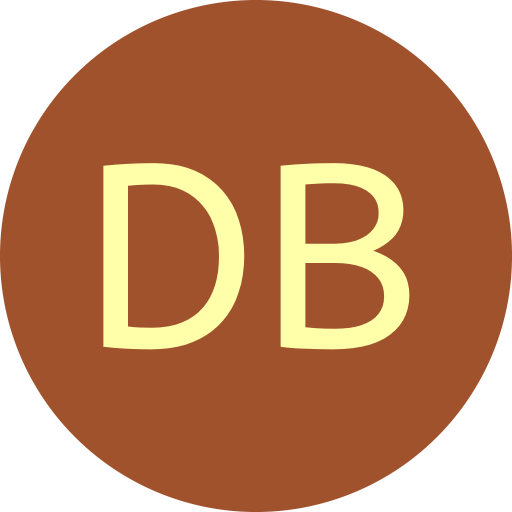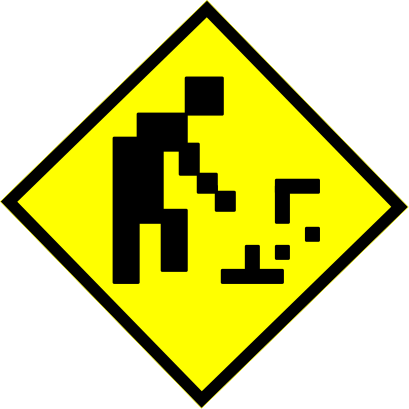#De Bruijn Sequence and Universal Cycle Constructions

Output
 Order $n$ Symbols $k$ 2 3 4 5 6 7 8 9 Cycle length $m$
Cut-down DB sequences

For some applications, a cycle of arbitrary length $1 \leq m \leq k^n$ is required such that every $k$-ary string of length $n$ appears at most once. For instance, consider trying to apply the de Bruijn Card Trick on a complete deck of 52 cards. We call such cycles cut-down de Bruijn sequences. They can also be thought of as universal cycles on a subset $\mathbf{S}$ of the $k$-ary strings of length $n$, where $|\mathbf{S}| = m$; however, the set $S$ is not known at the outset. The existence of such cycles for all $m$ was proved by Lempel [Lem71], and constructions for $k=2$ are given by Etzion [Etz86].

At a high-level, a cut-down DB sequence can be constructed in two steps:

1. Construct a main cycle $C$ that has length $m+n'$ where $0 \leq n' < n$.
2. Cut out small cycles whose length total $n'$ from $C$.
The secondary step is similar Hierholzer's cycle-joining algorithm on the de Bruijn graph, except we are removing cycles instead of merging them. Combining these two steps into an efficient symbol by symbol construction is not trivial.More details along with an implementation to appear soon.

Download source code

References

• [Etz86] T. Etzion. An algorithm for generating shift-register cycles. Theoret. Comput. Sci., 44(2):209-224, 1986.

• [Lem71] A. Lempel. $m$-ary closed sequences. Journal of Combinatorial Theory, Series A, 10(3):253-258, 1971.